Courses

# Previous Year Questions - Constructions Notes | EduRev

## Class 10 : Previous Year Questions - Constructions Notes | EduRev

The document Previous Year Questions - Constructions Notes | EduRev is a part of the Class 10 Course Mathematics (Maths) Class 10.
All you need of Class 10 at this link: Class 10

Short Answer Type Questions

Q.1. Draw two concentric circles of radii 2 cm and 5 cm. Take a point P on the outer circle and construct a pair of tangents PA and PB to the smaller circle. Measure PA.    [CBSE, Allahabad 2019]
Ans.  Steps of Construction: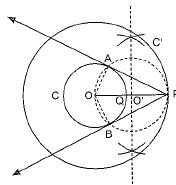(i) Draw concentric circles of radius OQ = 2 cm and OP = 5 cm having same centre O.
(ii) Mark these circles as C and C'.
(iii) Points O, Q and P lie on the same line.
(iv) Draw perpendicular bisector of OP, which intersects OP at O'.
(v) Take O' as centre, draw a circle of radius OO' which intersects circle C at points A and B.
(vi) Join PA and PB, these are the required tangents.
(vii) Length of these tangents are approx. 4.6 cm.
Justification:
Join OA and OB
OA ⊥ PA   [Radius ⊥ to tangent]
In right angled ΔOAP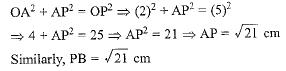A pair of equal tangents can be drawn to a circle from an external common point outside the circle.
∴ PA = PB

Q.2. Construct a triangle with sides 6 cm, 8 cm and 10 cm. Construct another triangle whose sides are 3/5 of the corresponding sides of original triangle.   [CBSE 2018 (C)]
Ans.
Steps of construction:
(a) Draw ΔABC, such that
AB = 8 cm, BC = 6 cm
AC = 10 cm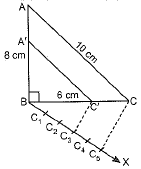(b) Draw any ray BX making an acute angle with BC on the side opposite to the vertex A.
(c) Draw 5 equal marks, C1, C2, C3, C4, C5 such that BC1 = C1C2 = C2C3 = C3C4 = C4C5
(d) Join CC5. Draw C3C' || C5C, where C' is any point on BC
(e) Draw C'A' || AC
(f) ΔA'BC' is the required triangle.

Q.3. A ladder 15 m long makes an angle of 60° with the wall. Find the height of the point where the ladder touches the wall.    [CBSE(F) 2017]
Ans.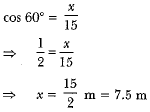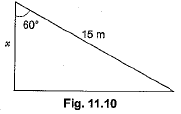Q.4. The ratio of the height of a tower and the length of its shadow on the ground is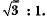What is the angle of elevation of the sun?    [CBSE Delhi 2017]
Ans.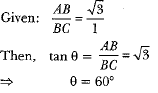Q.5. Draw a line segment of length 8 cm and divide it internally in the ratio 4 : 5.    [Delhi 2017]
Ans. Steps of construction:

(i) Draw a line segement AB of length 8 cm.
(ii) Draw any ray AX making an acute angle with AB.
(iii) Locate 9(i.e. 4+5) points A1, A2, A3, ... A9 on AX so that AA1 = A1A2 = ... A8A9
(iv) Join A9B.
(v) Through the point A4 draw a line parallel to A9B by making an angle equal to ∠AA9 B at A4 intersecting AB at point P.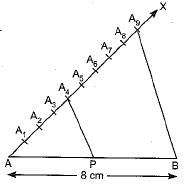Then AP : PR = 4 : 5

Q.6. If a tower 30 m high, casts a shadow 10 √3 m long on the ground, then what is the angle of elevation of the sun ?     [CBSE (Al) 2017]
Ans.
in ΔABC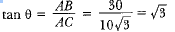⇒ tan θ = tan 60°
⇒ θ = 60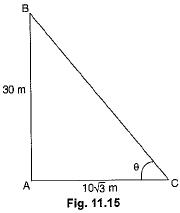Q.7. A moving boat is observed from the top of a 150 m high cliff moving away from the cliff. The angle of depression of the boat changes from 60° to 45° in 2 minutes. Find the speed of the boat in m/h.     [CBSE Delhi 2017]
Ans.
Let the speed of boat be x m/min
∴ CD = 2x
In ΔABC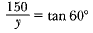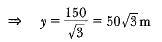In ΔABD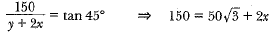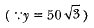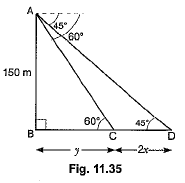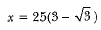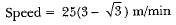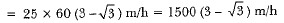Q.8. In Fig. 11.13, AB is a 6 m high pole and CD is a ladder inclined at an angle of 60° to the horizontal and reaches up to a point D of pole. If AD = 2.54 m, find the length of the ladder. (Use √3 =1.73)     [CBSE Delhi 2016]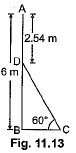Ans.
DB = (6 - 2.54)m = 3.46 m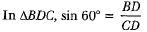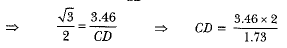∴ DC = 4m

Q.9. An observer, 1.7 m tall, is 20 √3 m away from a tower. The angle of elevation from the eye of observer to the top of tower is 30°. Find the height of tower.     [CBSE (F) 2016]
Ans.
Let AB be the height of tower and DE be the height of observer.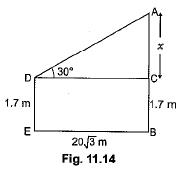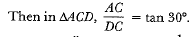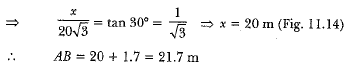Q.10. A vertical tower stands on a horizontal plane and is surmounted by a flagstaff of height 5 m. From a point on the ground the angles of elevation of the top and bottom of the flagstaff are 60° and 30° respectively. Find the height of the tower and the distance of the point from the tower. (Take √3 = 1.732)     [CBSE (F) 2016]
Ans.
Let height of tower be x m and distance of point from tower be y m.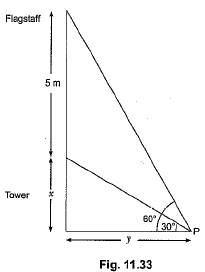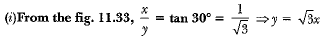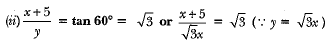⇒ x + 5 = 3x ⇒ x = 5/2 = 2.5
Height of tower = 2.5 m
Distance of point from tower = y = √3x
= (2.5 X 1.732) or 4.33 m

Q.11. Two men on either side of a 75 m high building and in line with base of building observe the angle of elevation of the top of the building as 30° and 60°. Find the distance between the two men. (Use √3 = 1.73)    [CBSE (F) 2016]
Ans.
ln ΔABM1 ,
Let AB be the building having height 75 m and the angles of elevation are 30° and 60° from the point M1 and M2 respectively;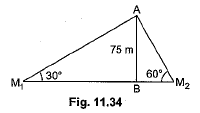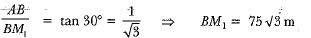In ΔABM2,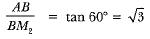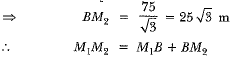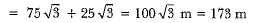∴ Distance between two men — 173 m.

Long Answer Type Questions

Q.1. Construct a ΔABC in which CA = 6 cm, AB = 5 cm and ΔBAC = 45°. Then construct a triangle whose sides are 3/5 of the corresponding sides of ΔABC.   [Delhi 2019]
Ans.
Steps of construction: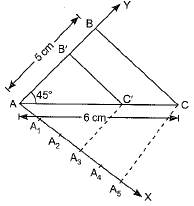(i) A line segment AC = 6 cm is drawn.
(ii) ∠CAB = 45° is constructed at A.
(iii) An arc of 5 cm radius to be drawn with A as centre, cutting AY at B.
(iv) B and C are joined. Then, ΔABC is constructed.
(v) An acute angle CAX is drawn below AC.
(vi) Points A1, A2, A3, A4 and A5 are taken on AX, such that AA1 = A3A2 = A2A3 = A3A4 = A4A
(vii) A5 and C are joined.
(viii) A3C' is drawn parallel to A5C, meeting AC at C' .
(ix) C'B' is drawn parallel to CB, meeting AB at B'.
(x) AB'C' is the required triangle similar to ΔABC whose sides a 3/5 of the corresponding sides of ΔABC.

Q.2. Two poles of equal heights are standing opposite to each other on either side of the road, which is 80 m wide. From a point between them on the road, the angles of elevation of the top of the poles are 60° and 30°, respectively. Find the height of the poles and the distances of the point from the poles.     [NCERT; CBSE 2019 (30/1/2)]
Ans.
Let AB and CD be two poles of equal height & metre and let P be any point between the poles, such that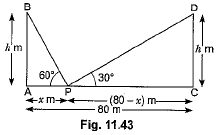∠APB = 60° and ∠DPC = 30°
The distance between two poles is 80m. (Given)
Let AP = x m, then PC = (80 - x) m
Now, in ΔAPB, we have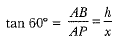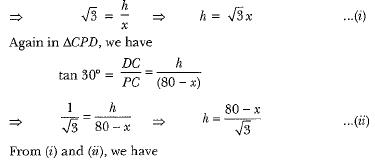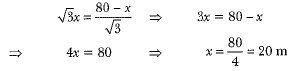Now, putting the value of x in equation (i), we have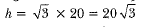Hence, the height of the pole is 20 √3 m and the distance of the point from first pole is 20 m and that of the second pole is 60 m.

Q.3. A boy standing on a horizontal plane finds a bird flying at a distance of 100 m from him at an elevation of 30°. A girl standing on the roof of 20 metre high building, finds the angle of elevation of the same bird to be 45°. Both the boy and the girl are on opposite sides of the bird. Find the distance o f bird from the girl. [Given √2 = 1.414]     [CBSE 2019 (30/5/1)]
Ans.
Let B be the position of bird. O and P be the positions of boy and girl respectively and PQ be the building
We have, ∠AOB = 30° and, ∠BPM = 45°
In ΔAOB, we have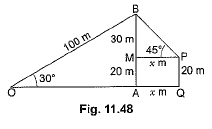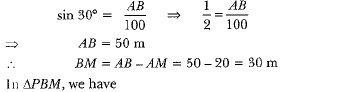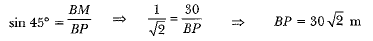Hence, distance of bird from girl is 30 √2 m.

Q.4. From the top of a 7 m high building, the angle of elevation of the top of a cable tower is 60° and the angle of depression of its foot is 45°. Determine the height of the tower.     [NCERT, CBSE Delhi 2017]
OR
From the top of a 7 m high building, the angle of elevation of the top of a tower is 60° and the angle of depression of its foot is 45°. Find the height of the tower. [Use √3 = 1.732]     [CBSE (F) 2017]
Ans.
Let PQ be the building of height 7 metres and AB be the cable tower. Now it is given that the angle of elevation of the top A of the tower observed from the top P of building is 60° and the angle of depression of the base B of the tower observed from P is 45°. (Fig. 11.38)
So, ∠APR = 60° and ∠QBP = 45°
Let QB = x m, AR = h m then, PR = x m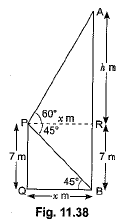Now, in ΔAPR, we have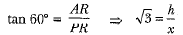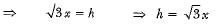...(i)
Again, in ΔPBQ we have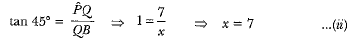Putting the value of x in equation (i), we have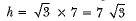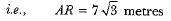So, the height of tower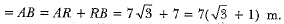Q.5. A straight highway leads to the foot of a tower. A man standing at the top of the tower observes a car at an angle of depression of 30°, which is approaching the foot of the tower with a uniform speed. Six seconds later, the angle of depression of the car is found to be 60°. Find the time taken by the car to reach the foot of the tower from this point.   [NCERT, CBSE Delhi 2017 (C)]
Ans. Let OA be the tower of height h, and P be the initial position of the car when the angle of depression is 30°.
After 6 seconds, the car reaches to Q such that the angle of depression at Q is 60°. Let the speed of the car be v metre per second. Then,
PQ = 6v     (∴ Distance = speed x time)
and let the car take i seconds to reach the tower OA from Q (Fig. 11.41). Then, OQ = vt metres.
Now, in ΔAQO, we have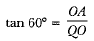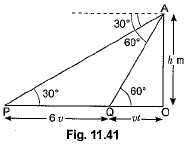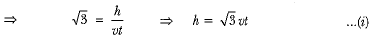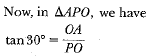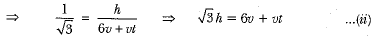Now, substituting the value of h from (i) into (ii), we have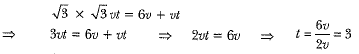Hence, the car will reach die tower from Q in 3 seconds.

Q.6. From the top of a tower, 100 m high, a man observes two cars on the opposite sides of the tower and in same straight line with its base, with angles of depression 30° and 45°. Find the distance between the cars.  [Take √3= 1.732]      [CBSE (AI) 2017]
Ans.
Let AO be the tower of height 100 m. Car B and Car C are in opposite direction and at distance of x m and y m respectively.
In ΔABO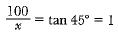⇒ x = 100   ...(i)
In ΔACO,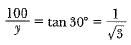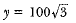...(ii)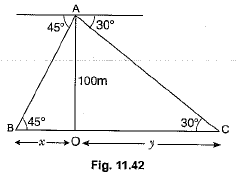Distance between the cars - x + y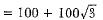[From equation (i) and (ii)]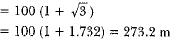Q.7. The shadow of a tower at a time is three times as long as its shadow when the angle of elevation of the sun is 60°. Find the angle of elevation of the sun at the time of the longer shadow.     [CBSE(F) 2017]
Ans. Let AB be the flagstaff and BC be the length of its shadow when the Sun rays meet the ground at an angle of 60°. Let 0 be the angle between the Sun rays and the ground when the length of the shadow of the flagstaff is BD. Let h be the height of the flagstaff (Fig. 11.52).
Let BC = x  ∴ BD = 3x and CD = 2x
In ΔABC, we have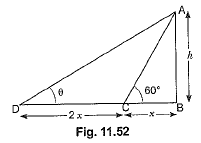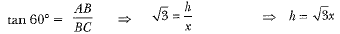In ΔADB, we have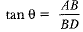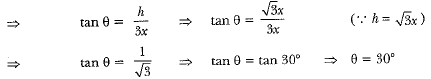Q.8. Construct a triangle ABC with side BC = 7 cm, ∠B = 45°, ∠A = 105°. Then construct another triangle whose sides are 3/4 times the corresponding sides of the ΔABC.    [AI 2017]
Ans.
In ΔABC,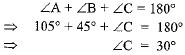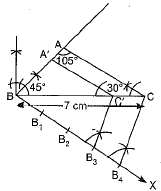Steps of Construction:
1. Draw a line segment BC = 7 cm. At the point B, draw ∠B = 45° and at C, draw ∠C = 30° and get ΔABC.
2. Draw an acute CBX on the base BC at the point B (In downward direction). Mark the ray BX with four equal points B1, B2, B3 and B4 such that BB1 = B1B2 = B2B3 = B3B4.
3. Join B4 to C. Draw B3C'||B4C.
4. At C', draw C'A'||AC.
5. ΔABC' is the required triangle.

Q.9.  Draw an isosceles triangle ABC in which the base BC is 8 cm long and its altitude AD through A is 4 cm long. Then draw another triangle whose sides are 2/3 of the corresponding sides of the ΔABC.    [AI 2017 (C)]
Ans.
Steps of construction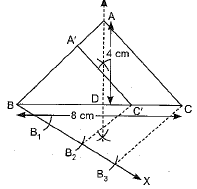1. Draw an isosceles ΔABC in which BC = 8 cm, AD = 4 cm and AB = AC
2. Draw an acute angle CBX below BC at point B.
3. Draw three equal marks B1, B2, B3 on BX, such that
BB1 = B1B2 = B2B
4. Join B3C, draw B2C' || B3C.
5. At point C', draw C'A' || AC.
6. ΔA'BC' is the required isosceles triangle.

Q.10. From the top of a hill, the angles of depression of two consecutive kilometre stones due east are found to be 45° and 30° respectively. Find the height of the hill.     [CBSE (E) 2017]
Ans.
Let the height of the hill be h m, C and D are two consecutive stones having distance 1000 m between them and 4C = x m.
In ΔABC,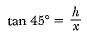⇒ x = h   ...(i)
In ΔABD,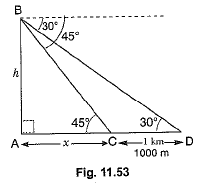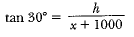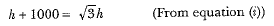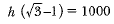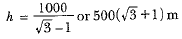Hence, the height of the hill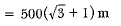Offer running on EduRev: Apply code STAYHOME200 to get INR 200 off on our premium plan EduRev Infinity!

## Mathematics (Maths) Class 10

51 videos|346 docs|103 tests

,

,

,

,

,

,

,

,

,

,

,

,

,

,

,

,

,

,

,

,

,

;